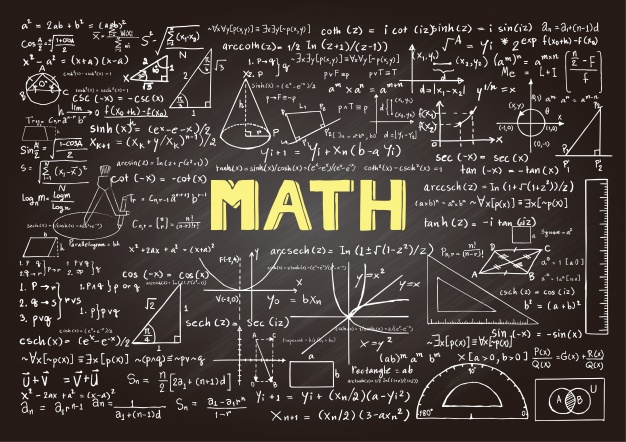# Everything You May Not Know About Discrete MathRaise the subject of discrete math in a group and, sad to say, you will be in a monologue. Nobody wants to hear about this subject. It’s like a tattoo not to be associated with at all costs.

But,

## Is Discrete Math Hard?

The answer is No, and there are many supporting arguments to this. Just like linear algebra and calculus, which are taught in high school, discrete math too, can easily be understood.

It merely deals with understanding basically what mathematics is and the working of mathematical proofs and definitions.

Is it getting simplified now?

Discrete math is applied majorly in computer science (digital computers) because of its distinct nature – numbers are not continuous.

Naturally, it is calculus elaborated a bit in detail. Not some complicated rocket science as many would have thought. Is discrete math then harder than calculus? Not at all.

Are you ready to explore the reasons why discrete math is not hard?

## Discrete Math is Not Hard: Here Are The Reasons

### 1. It Is Practical

Theoretical classes seem dull and of no use, right? I mean, can you apply the linear algebra theoretical knowledge in real-life situations daily? Many students are thus discouraged with this.

With discrete math, however, a student can be able to apply it to everyday intriguing, and exciting challenges. Doesn’t this sound fascinating now?

That’s not the end of the story.

### 2. It Enables You Think Mathematically And Prove This

Memorizing a long list of formulas is the order of the day in calculus or algebra. Do you still remember the equations you learned in your first year or sophomore now that you are in your final year? Hectic, right?

Nevertheless, discrete math introduces a concept so important, yet lacking in the other mathematical concepts. That is, thinking creatively in a precise manner.

Creativity, at its best, will enable you to solve problems in discrete math as opposed to the rigid formulas in calculus and linear algebra.

Is discrete math harder than calculus now?

What will make you stand out in a contest or interview? Is it that neatly combed hair with a three-piece suit directly from the tailor’s shop? No.

It’s the unique quality that you have above all the other applicants or competitors. Discrete math does justice to this.

Topics such as probability and counting in discrete math will broaden the students’ mindset in tackling any other mathematical problem. These topics are the core of mathematics. There will be two or three questions on the same in case of a contest.

### 4. It Is Exciting

Who else wants to sit down for an hour or so to listen or watching a math lecturer scribble down lines of numbers and signs unfamiliar to them?

Well, geometry and linear algebra does just this, but not discrete math. The practicality of discrete math makes it interactive and, ultimately, fun!

Number theory, probability, and counting give room for the teacher and students to mingle and excitingly solve problems.

### 5. Like Any Other Mathematical Concept, Attitude Matters

According to statistics, most students’ fear’ or ‘hate’ mathematics as a whole. Discrete math thus falls victim to this as well.

If you get a captivating and inspirational mathematics teacher, you will love the subject. Every concept will be simplified, and you will comfortably sail like a boat on a calm, warm, sunny day in a sea.

The case might be worse if the teacher is hard on you. Interest may slowly fade away, and you will develop a negative attitude towards the subject.

## Bringing It Back Home

Everything starts in mind. Once you develop a positive and enthusiastic attitude towards discrete math, it will be like having your favorite ice cream at a candy shop. Relaxing and entertaining.

Like any other journey, start with a step. The basics of discrete math should be handled first while carefully advancing to the complex ones. Look for a tutor or a friend who is better off and together, solve it.

Is discrete math important for computer science? Yes, it is. So, if you are looking forward to pursuing a course in this line, then let discrete math be your best friend from now henceforth.

Take a step towards facing it, and in the end, you will comfortably say, ‘I made it!’

How hard is discrete math now? Simplified, right? (I can see your face brightening up now). It is cheap, and if you need any assignment help on discrete math problems, our team is ready and willing to do so.